# NCERT Solutions class-11 Maths Exercise 16.1## myCBSEguide App

CBSE, NCERT, JEE Main, NEET-UG, NDA, Exam Papers, Question Bank, NCERT Solutions, Exemplars, Revision Notes, Free Videos, MCQ Tests & more.

Exercise 16.1

In each of the following exercises 1 to 7, describe the sample space for the indicated experiment.

1. A coin is tossed three times.

Ans. S = {HHH, HHT, HTH, THH, TTH, HTT, TTT}

2. A die is thrown two times.

Ans. S = {(1, 1), (1, 2), (1, 3), (1, 4), (1, 5), (1, 6)

(2, 1), (2, 2), (2, 3), (2, 4), (2, 5), (2, 6)

(3, 1), (3, 2), (3, 3), (3, 4), (3, 5), (3, 6)

(4, 1), (4, 2), (4, 3), (4, 4), (4, 5), (4, 6)

(5, 1), (5, 2), (5, 3), (5, 4), (5, 5), (5, 6)

(6, 1), (6, 2), (6, 3), (6, 4), (6, 5), (6, 6)}

3. A coin is tossed four times.

Ans. S = {HHHH, HHHT, HHTH, HTHH, THHH, HHTT, HTHT, THHT, HTTH, THTH, TTHH, TTTH,

TTHT, THTT, HTTT, TTTT}

4. A coin is tossed and a die is thrown.

Ans. When a coin is tossed, then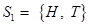When a die is thrown then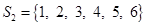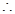S = {H1, H2, H3, H4, H5, H6, T1, T2, T3, T4, T5, T6}

5. A coin is tossed and then a die is rolled only in case a head is shown on the coin.

Ans. When a coin is tossed, thenWhen a die is rolled thenS = {H1, H2, H3, H4, H5, H6, T}

6. 2 boys and 2 girls are in a Room X and 1 boy and 3 girls in Room Y. Specify the sample space for the experiment in which a room is selected and then a person.

Ans. Let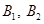denote the boys and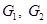girls in room X and B1 denotes the boy and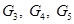girls in room Y, then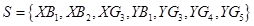7. One die of red colour, one of white colour and one of blue colour are placed in a bag. One die is selected at random and rolled, its colour and the number on its upper most face is noted. Describe the sample space.

Ans. The three are R, W and B, then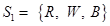A die is rolled thenS = {R1, R2, R3, R4, R5, R6, W1, W2, W3, W4, W5, W6, B1, B2, B3, B4, B5, B6}

8. An experiment consists of recording boy-girl composition of families with 2 children.

(i) What is the sample space if we are interested in knowing whether it is a boy or girl in the order of their births?

(ii) What is the sample space if we are interested in the number of girls in the family?

Ans. There are two children in a family which are to be boys or girls.

(i)S = {BB, BG, GB, GG}

(ii) When the outcome is BB, then number of girls is 0.

When the outcome is BG, then number of girls is 1.

When the outcome is GB, then number of girls is 1.

When the outcome is GG, then number of girls is 2.S = {0, 1, 2}

9. A box contains 1 red and 3 identical white balls. Two balls are drawn at random in succession without replacement. Write the sample space for this experiment.

Ans. Let the four balls in the box are R, W, W W.

Two balls are drawn without replacement, then S = {RW, WR, WW}

10. An experiment consists of tossing a coin and then throwing it second time if a head occurs. If a tail occurs on the first toss, then a die is rolled once. Find the sample space.

Ans. A coin is tossed, thenA coin is tossed again, then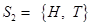A die is rolled then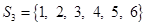S = {HH, HT, T1, T2, T3, T4, T5, T6}

11. Suppose 3 bulbs are selected at random from a lot. Each bulb is tested and classified as defective (D) or non-defective (N). Write the sample space of this experiment.

Ans. Let defective bulb be denoted by D and non-defective bulb be denoted by N.S = {DDD, DDN, DND, NDD, DNN, NDN, NND, NNN}

12. A coin is tossed. If the outcome is a head, a die is thrown. If the die shows up an even number, the die is thrown again. What is the sample space for the experiment?

Ans. When a coin is tossed, thenWhen the coin shows T, then there is no action.

When the coin shows H, a die is thrown thenWhen the die shows {1, 3, 5}, then there is no action.

When the die shows {2, 4, 6}, then a die is thrown again.S = {T, H1, H3, H5, H21, H22, H23, H24, H25, H26, H41, H42, H43, H44, H45, H46, H61,

H62, H63, H64, H65, H66}

13. The number 1, 2, 3 and 4 are written separately on four slips of paper. The slips are put in a box and mixed thoroughly. A person draws two slips from the box, one after the other without replacement. Describe the sample space for the experiment.

Ans. When two slips are drawn from the box without replacement then the sample space is

S = {(1, 2), (1, 3), (1, 4), (2, 1), (2, 3), (2, 4), (3, 1), (3, 2), (3, 4), (4, 1), (4, 2), (4, 3)}

14. An experiment consists of rolling a die and then tossing a coin once if the number on the die is even. If the numbers on the die is odd, then coin is tossed twice. Write the sample space for this experiment.

Ans. When a die is thrown then S ={1, 2, 3, 4, 5, 6}

When the number on die is {2, 4, 6}

15. A coin is tossed. If it shows a tail, we draw a ball from a box which contains 2 red and 3 black balls. If it shows head, we throw a die. Find the sample space for this experiment.

Ans. When a coin is tossed, thenWhen the coin shows T, then draw a ball from a box.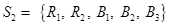When the coin shows H, then a die is thrown then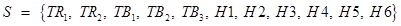16. A die is thrown repeatedly until a six comes up. What is the sample space for this experiment?

Ans. A die is thrown, when 6 comes up in first draw then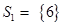A die is thrown, when 6 comes up in second draw then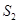= {(1, 6), (2, 6), (3, 6), (4, 6), (5, 6)}

And so on.S = {(6), (1, 6), (2, 6), (3, 6), (4, 6), (5, 6), (1, 2, 6), ………. (1, 5, 6), (2, 1, 6), …… (5, 2, 6)…}